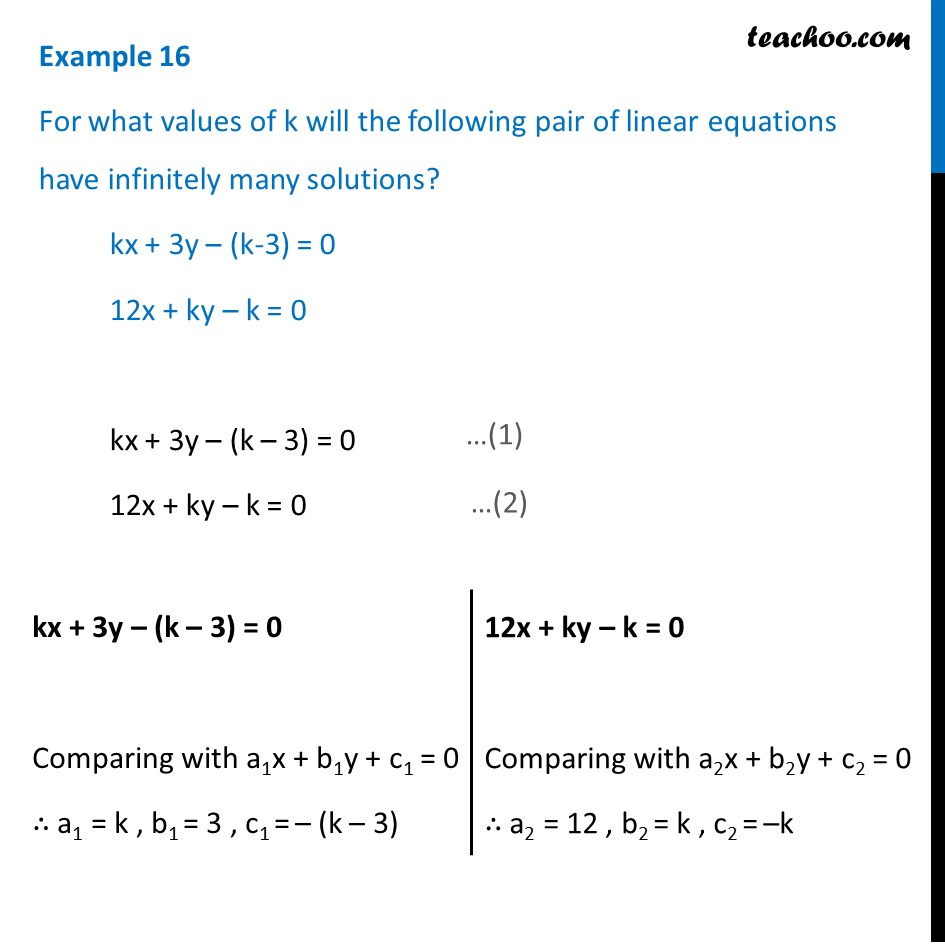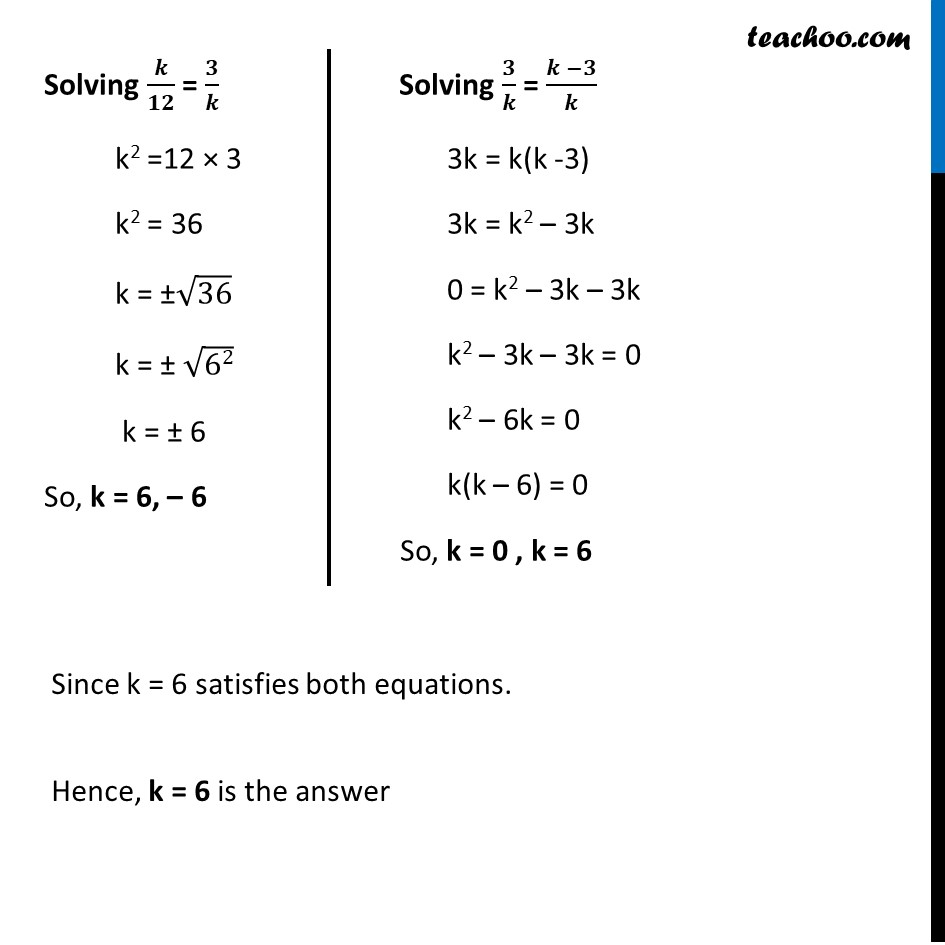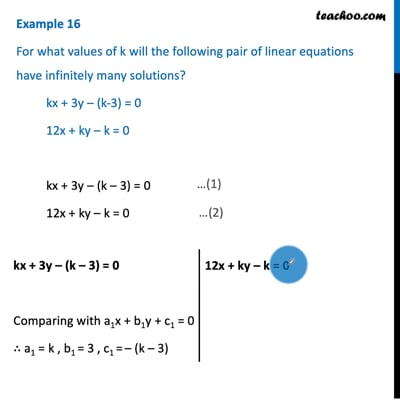Examples

Chapter 3 Class 10 Pair of Linear Equations in Two Variables
Serial order wiseThis video is only available for Teachoo black users

Solve all your doubts with Teachoo Black (new monthly pack available now!)

### Transcript

Example 16 For what values of k will the following pair of linear equations have infinitely many solutions? kx + 3y – (k-3) = 0 12x + ky – k = 0 kx + 3y – (k – 3) = 0 12x + ky – k = 0 kx + 3y – (k – 3) = 0 Comparing with a1x + b1y + c1 = 0 ∴ a1 = k , b1 = 3 , c1 = – (k – 3) 12x + ky – k = 0 Comparing with a2x + b2y + c2 = 0 ∴ a2 = 12 , b2 = k , c2 = –k Since equation has infinite number of solutions So, 𝑎1/𝑎2 = 𝑏1/𝑏2 = 𝑐1/𝑐2 Putting values 𝑘/12 = 3/𝑘 = (−(𝑘 − 3))/(−𝑘) 𝑘/12 = 3/𝑘 = (𝑘 − 3)/𝑘 Solving 𝒌/𝟏𝟐 = 𝟑/𝒌 k2 =12 × 3 k2 = 36 k = ±√36 k = ± √62 k = ± 6 So, k = 6, – 6 Solving 𝟑/𝒌 = (𝒌 −𝟑)/𝒌 3k = k(k -3) 3k = k2 – 3k 0 = k2 – 3k – 3k k2 – 3k – 3k = 0 k2 – 6k = 0 k(k – 6) = 0 So, k = 0 , k = 6 Since k = 6 satisfies both equations. Hence, k = 6 is the answer